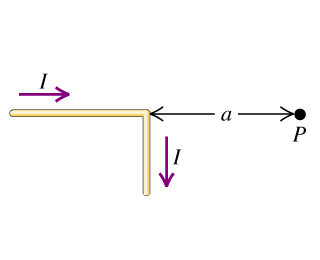# Problem: The wire shown in the figure (Figure 1) is infinitely long and carries a current.A. Calculate the magnitude of the magnetic field that this current produces at point P.B. Find the direction of the magnetic field that this current produces at point P.

###### FREE Expert Solution

From Biot-Savart Law:

$\overline{)\begin{array}{rcl}\mathbf{d}{\stackrel{\mathbf{⇀}}{\mathbf{B}}}_{\mathbf{h}\mathbf{o}\mathbf{r}\mathbf{i}\mathbf{z}\mathbf{o}\mathbf{n}\mathbf{t}\mathbf{a}\mathbf{l}}& {\mathbf{=}}& \mathbf{\left(}\frac{{\mathbf{\mu }}_{\mathbf{0}}}{\mathbf{4}\mathbf{\pi }}\mathbf{\right)}\mathbf{\left(}\frac{\mathbf{i}\mathbf{d}\stackrel{\mathbf{⇀}}{\mathbf{l}}\mathbf{×}\stackrel{\mathbf{^}}{\mathbf{r}}}{{\mathbf{r}}^{\mathbf{2}}}\mathbf{\right)}\\ & {\mathbf{=}}& \frac{{\mathbf{\mu }}_{\mathbf{0}}}{\mathbf{4}\mathbf{\pi }}\frac{\mathbf{i}\mathbf{d}\stackrel{\mathbf{⇀}}{\mathbf{l}}\mathbf{s}\mathbf{i}\mathbf{n}\mathbf{\theta }}{{\mathbf{r}}^{\mathbf{2}}}\end{array}}$

The magnetic field due to the infinite current-carrying wire is:

$\overline{)\stackrel{\mathbf{⇀}}{\mathbf{B}}{\mathbf{=}}\frac{{\mathbf{\mu }}_{\mathbf{0}}\mathbf{i}}{\mathbf{2}\mathbf{\pi }\mathbf{r}}}$

A.

For the horizontal wire, θ = 0°

Since sin(0°) = 0

dBhorizontal = 0

96% (240 ratings)###### Problem Details

The wire shown in the figure (Figure 1) is infinitely long and carries a current.A. Calculate the magnitude of the magnetic field that this current produces at point P.

B. Find the direction of the magnetic field that this current produces at point P.

Frequently Asked Questions

What scientific concept do you need to know in order to solve this problem?

Our tutors have indicated that to solve this problem you will need to apply the Biot-Savart Law (Calculus) concept. You can view video lessons to learn Biot-Savart Law (Calculus). Or if you need more Biot-Savart Law (Calculus) practice, you can also practice Biot-Savart Law (Calculus) practice problems.

What professor is this problem relevant for?

Based on our data, we think this problem is relevant for Professor Jerousek's class at UCF.